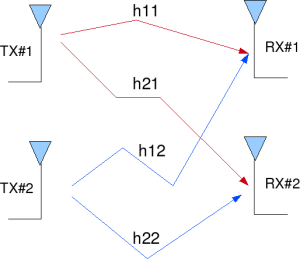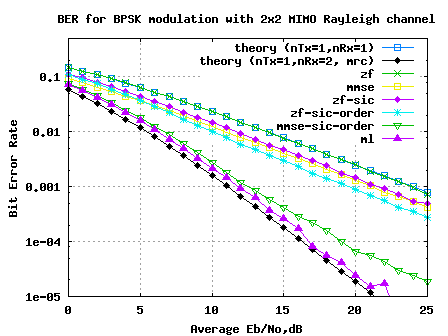# Six equalizers for V-BLAST

In the past, we had discussed several posts on two transmit two receive MIMO communication, where the transmission was based on V-BLAST. The details about V-BLAST can be read from the landmark paper V-BLAST: An architeture for realizing very high data rates over the rich scattering wireless channel – P. W. Wolniansky, G. J. Foschini, G. D. Golden, R. A. Valenzuela. We will assume that the channel is a flat fading Rayleigh multipath channel and the modulation is BPSK.Figure: 2 transmit 2 receive MIMO channel

## V-BLAST transmission for 2×2 MIMO channel

In a 2×2 MIMO channel, probable usage of the available 2 transmit antennas can be as follows:

1. Consider that we have a transmission sequence, for example

2. In normal transmission, we will be sending in the first time slot, in the second time slot, and so on.

3. However, as we now have 2 transmit antennas, we may group the symbols into groups of two. In the first time slot, send and from the first and second antenna. In second time slot, send and from the first and second antenna, send and in the third time slot and so on.

4. Notice that as we are grouping two symbols and sending them in one time slot, we need only time slots to complete the transmission – data rate is doubled !5. This forms the simple explanation of a probable MIMO transmission scheme with 2 transmit antennas and 2 receive antennas.

## Other Assumptions

1. The channel is flat fading – In simple terms, it means that the multipath channel has only one tap. So, the convolution operation reduces to a simple multiplication. For a more rigorous discussion on flat fading and frequency selective fading, may I urge you to review Chapter 15.3 Signal Time-Spreading from [DIGITAL COMMUNICATIONS: SKLAR]2. The channel experience by each transmit antenna is independent from the channel experienced by other transmit antennas.

3. For the transmit antenna to receive antenna, each transmitted symbol gets multiplied by a randomly varying complex number . As the channel under consideration is a Rayleigh channel, the real and imaginary parts of are Gaussian distributed having mean and variance .

4. The channel experienced between each transmit to the receive antenna is independent and randomly varying in time.

5. On the receive antenna, the noise has the Gaussian probability density function with

with and .

7. The channel is known at the receiver.

## System Model

.

.

where

, are the received symbol on the first and second antenna respectively,

is the channel from transmit antenna to receive antenna,

is the channel from transmit antenna to receive antenna,

is the channel from transmit antenna to receive antenna,

is the channel from transmit antenna to receive antenna,

, are the transmitted symbols and

is the noise on receive antennas.

We assume that the receiver knows , , and . The receiver also knows and . The unknown s are and . Two equations and two unknowns. Can we solve it? Answer is YES.For convenience, the above equation can be represented in matrix notation as follows:

.

Equivalently,

### MIMO with Zero Forcing Equalization

The zero forcing approach tries to find a matrix which satisfies . The Zero Forcing (ZF) linear detector for meeting this constraint is given by,

.

### MIMO with MMSE Equalization

The Minimum Mean Square Error (MMSE) approach tries to find a coefficient which minimizes the criterion,

.

Solving,

.

### Zero Forcing Equalization with Successive Interference Cancellation

Using the Zero Forcing (ZF) equalization approach described above, the receiver can obtain an estimate of the two transmitted symbols , , i.e.

.

Take one of the estimated symbols (for example ) and subtract its effect from the received vector and , i.e.

.

Expressing in matrix notation,

,

The above equation is same as equation obtained for receive diversity case. Optimal way of combining the information from multiple copies of the received symbols in receive diversity case is to apply Maximal Ratio Combining (MRC).

The equalized symbol is,

.

This forms the simple explanation for Zero Forcing Equalizer with Successive Interference Cancellation (ZF-SIC) approach.

### Zero Forcing Equalization with Optimally ordered Successive Interference Cancellation

In classical Successive Interference Cancellation, the receiver arbitrarily takes one of the estimated symbols, and subtract its effect from the received symbol and . However, we can have more intelligence in choosing whether we should subtract the effect of first or first. To make that decision, let us find out the transmit symbol (after multiplication with the channel) which came at higher power at the receiver. The received power at the both the antennas corresponding to the transmitted symbol is,

.

The received power at the both the antennas corresponding to the transmitted symbol is,

.

If then the receiver decides to remove the effect of from the received vector and and then re-estimate . Else if the receiver decides to subtract effect of from the received vector and , and then re-estimate .

### MMSE equalization with optimaly ordered Successive Interference Cancellation

Using the Minimum Mean Square Error (MMSE) equalization, the receiver can obtain an estimate of the two transmitted symbols , , i.e.

.

If then the receiver decides to remove the effect of from the received vector and . Else if the receiver decides to subtract effect of from the received vector and , and then re-estimate .

Once the effect of either or is removed, the new channel becomes a one transmit antenna, 2 receive antenna case and the symbol on the other spatial dimension can be optimally equalized by Maximal Ratio Combining (MRC).

### MIMO with ML equalization

The Maximum Likelihood receiver tries to find which minimizes,

Since the modulation is BPSK, the possible values of is +1 or -1 Similarly also take values +1 or -1. So, to find the Maximum Likelihood solution, we need to find the minimum from the all four combinations of and .

The estimate of the transmit symbol is chosen based on the minimum value from the above four values i.e

if the minimum is ,

if the minimum is ,

if the minimum is and

if the minimum is .

## Simulation Results

The plot embedded below captures BER for 2 transmit 2 receive MIMO V-BLAST ttansmission/reception for BPSK modulation in a flat fading independent Rayleigh channel, for the different equalizer structures discussed above.Figure: BER plot for 2 transmit 2 receive MIMO channel for BPSK modulation

## Observations

1. The BER curve with ZF equalization for 2×2 MIMO channel is identical to BER plot for 1 transmit 1 receive system

2. Ordered variant of successive interference cancellation shows better performance than the simple successive interference cancellation

3. MMSE equalization with ordered successive interference cancellation provides performance which is slightly poorer than ML.

4. With ML equalization, we come close to the performance of 1 transmit 2 receive MRC case. We gain both throughput gain and diversity gain.

## Reference

[DIG-COMM-BARRY-LEE-MESSERSCHMITT] Digital Communication: Third Edition, by John R. Barry, Edward A. Lee, David G. Messerschmitt